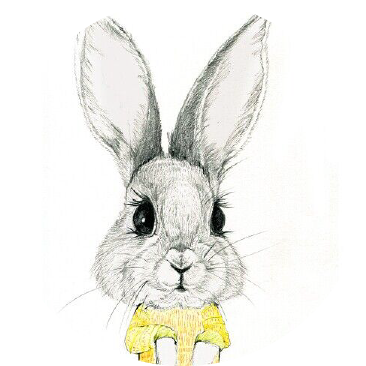#### 一、贝叶斯统计学框架

##### 2、先验概率

$$\displaystyle p(\beta)$$

##### 3、样本的产生与似然函数

1、上帝从先验分布 $\displaystyle p(\beta)$中选了一个 $\displaystyle \beta_k$我们人类不知道，但是可以$设想$。
2、从总体分布 $\displaystyle p(x\mid\beta_k)$产生一个样本 $\displaystyle \boldsymbol{x}=[x_1,x_2,…,x_n]$，这是具体的，我们人类能看到的。(按照频率学派观点，这里我们蕴含了 $\displaystyle x_i$是随机变量，且独立同分布的，贝叶斯认为这不需要，不过通常情况下，我们是使用IID，因为这样方便。)：
$$\mathrm{L}(\beta_k)=p(\boldsymbol{x}\mid \beta_k)=\prod_{i=1}^{n}p(x_i\mid\beta_k)$$我们称 $\displaystyle \mathrm{L}(\beta_i)$为似然函数，它综合了总体信息和样本信息。

##### 4、样本与参数的联合分布

$$p(\boldsymbol{x},\beta)=p(\boldsymbol{x}\mid \beta)p(\beta)$$

##### 5、贝叶斯推断

1、先分解： $\displaystyle p(\boldsymbol{x},\beta)=p(\beta\mid\boldsymbol{x})p(\boldsymbol{x})$。
2、其中 $\displaystyle p(\boldsymbol{x})=\int_{\mathcal{B}}p(\boldsymbol{x},\beta)\mathrm{d}\beta=\int_{\mathcal{B}} p(\boldsymbol{x}\mid \beta)p(\beta) \mathrm{d}\beta$ ，它与$\beta$无关，或者说 $\displaystyle p(\boldsymbol{x})$不含 $\displaystyle \beta$的任何信息。因此能用来推断的仅仅是条件分布：
$$p(\beta\mid\boldsymbol{x})=\frac{p(\boldsymbol{x},\beta)}{p(\boldsymbol{x})}=\frac{p(\boldsymbol{x}\mid\beta)p(\beta)}{\displaystyle\int_{\mathcal{B}} p(\boldsymbol{x}\mid \beta)p(\beta) \mathrm{d}\beta}$$这就是贝叶斯公式的概率密度函数形式。 $\displaystyle p(\beta\mid\boldsymbol{x})$史称后验分布，它集中了总体、样本、先验的一切信息，又排除了一切与 $\displaystyle \beta$无关的信息之后得到的结果。所以基于后验分布 $\displaystyle p(\beta\mid\boldsymbol{x})$对 $\displaystyle \beta$进行统计推断是更为有效，也是最合理的。

$$p(\beta_k\mid\boldsymbol{x})=\frac{p(\boldsymbol{x},\beta_k)}{p(\boldsymbol{x})}=\frac{p(\boldsymbol{x}\mid\beta_k)p(\beta_k)}\sum_{\beta\in \mathcal{B}}p(\boldsymbol{x}\mid \beta)p(\beta)$$

$$\text{posterior}\propto\text{likeihood}\times\text{prior}$$

$$p(\beta\mid\boldsymbol{x})\propto p(\boldsymbol{x}\mid \beta)p(\beta)$$

##### 6、贝叶斯统计分析的关键问题：

1、确定先验分布： $\displaystyle p(\beta)$
2、求联合分布： $\displaystyle p(\boldsymbol{x},\beta)$
3、求后验分布： $\displaystyle p(\beta\mid \boldsymbol{x})$

#### 二、一个精彩的入门例子

[例子1.0] 为了提高相亲的成功率，小美考虑打扮一下自己，于是决定买一件羊绒大衣。预计要花费2000块。但是对相亲效果的影响，闺蜜们有2种意见：

$\displaystyle \beta_1$：相亲成功率提高到90%
$\displaystyle \beta_2$：相亲成功率提高到70%

$$p(\beta_1)=0.4,\quad p(\beta_2)=0.6$$小美不想花冤枉钱，于是她做了一个测试：把自己看中大衣，ps一下照片，给5个男性朋友看，结果：
$$A: 5个男性朋友都认为小美更漂亮了$$小美对测试很满意，于是她改变了看法，由二项分布知：$$p(A\mid \beta_1)=0.9^5=0.590,\quad p(A\mid\beta_2)=0.7^5=0.168$$

$$p(\beta_1\mid A)=\frac{p(A\mid \beta_1)p(\beta_1)}{p(A)}=0.7,\quad p(\beta_2)=\frac{p(A\mid \beta_2)p(\beta_2)}{p(A)}=0.3$$这个概率综合了小美主观和实验的结果获得，要比小美之前认识的更有吸引力，更贴近实际。

$$p(\beta_1\mid B)=\frac{p(B\mid \beta_1)p(\beta_1)}{p(B)}=0.883,\quad p(\beta_2)=\frac{p(B\mid \beta_2)p(\beta_2)}{p(B)}=0.117$$小美经过两次测试，$\displaystyle \beta_1(相亲成功率提高到90\%)$的概率上升到了0.883，可以下决心买了。

#### 三、共轭先验分布

$\displaystyle \pi(\boldsymbol{\beta}\mid \boldsymbol{X})=\frac{h(\boldsymbol{X},\boldsymbol{\beta})}{m(\boldsymbol{X})}=\frac{p(\boldsymbol{X}\mid\boldsymbol{\beta})\pi(\boldsymbol{\beta})}{\displaystyle\int_{\mathcal{B}}p(\boldsymbol{X}\mid\boldsymbol{\beta})\pi(\boldsymbol{\beta})\mathrm{d}\boldsymbol{\beta}}$

##### 2、一维随机变量共轭先验分布例子
###### 1、方差已知下，一维高斯分布均值的先验分布是高斯分布。

1、样本似然函数 $\displaystyle p(\mathcal{D}\mid\mu)=p(\boldsymbol{x}\mid\mu)=\prod_{i=1}^{n}p(x_i\mid\mu)=(2\pi\sigma^2)^{-n/2}\exp\left[-\frac{1}{2}(\boldsymbol{x}-\mu\boldsymbol{I})^\text{T}\sigma^{-2}(\boldsymbol{x}-\mu\boldsymbol{I})\right]$
2、取 $\displaystyle \mu\sim\mathcal{N}(\mu\mid \bar{\mu},\delta)$为先验分布，其中 $\displaystyle \bar{\mu},\delta$是已知的。接下来我们将看到它是共轭的。
3、于是有联合分布：
$\displaystyle p(\mathcal{D},\mu)=p(\mathcal{D}\mid \mu)p(\mu)=\frac{1}{(2\pi)^{\frac{n+1}{2}}\sigma^{-\frac{n}{2}}\delta^{-1}}\exp\left[-\frac{1}{2}[(\boldsymbol{x}-\mu\boldsymbol{I})^\text{T}\sigma^{-2}(\boldsymbol{x}-\mu\boldsymbol{I})+(\mu-\bar{\mu})^2\delta^{-2}]\right]$
4、应用自然语言的贝叶斯定理，我们有后验分布：
\begin{align} p(\mu\mid\mathcal{D})=\propto& p(\boldsymbol{x}\mid \mu)p(\mu)\propto \exp\left[-\frac{1}{2}[(\boldsymbol{x}-\mu\boldsymbol{I})^\text{T}\sigma^{-2}(\boldsymbol{x}-\mu\boldsymbol{I})+(\mu-\bar{\mu})^2\delta^{-2}]\right] \\ \propto&\exp\left[-\frac{1}{2}\left((\frac{n}{\sigma^2}+\frac{1}{\delta^2})\mu^2-2(\frac{\boldsymbol{x}^\text{T}\boldsymbol{I}}{\sigma^2}+\frac{\bar{\mu}}{\delta^2})\mu+\frac{\boldsymbol{x}^\text{T}\boldsymbol{x}}{\sigma^2}+\frac{\bar{\mu}}{\delta^2}\right)\right]\\ \propto &\exp\left[-\frac{1}{2}(A\mu^2-2B\mu+C)\right]\\ \propto &\exp\left[-\frac{1}{2}\frac{(\mu^2-B/A)^2}{A^{-1}}\right] \end{align}

$$\displaystyle p(\mu\mid\mathcal{D})=p(\mu\mid\boldsymbol{x})=\frac{1}{(2\pi A^{-1})^{\frac{1}{2}}}\exp\left[-\frac{1}{2}\frac{(\mu^2-B/A)^2}{A^{-1}}\right]$$

$$\mu\mid\mathcal{D}\sim\mathcal{N}(\mu\mid \frac{B}{A},A^{-1})$$

$\displaystyle \frac{B}{A}=\frac{\delta^2}{\bar{\sigma}^2+\delta^2}\bar{x}+\frac{\bar{\sigma}^2}{\bar{\sigma}^2+\delta^2}\bar{\mu}=\lambda\bar{x}+(1-\lambda)\bar{\mu}$
$\displaystyle \frac{1}{\delta^2}=\frac{1}{\bar{\sigma}^2}+\frac{1}{\delta^2}$

##### 3、若干技巧总结

1、贝叶斯分析非常依赖于去求后验分布,如果按照定理，分母有一个积分，事实上它是一个数。于是我们经常应用$$\text{posterior}\propto\text{likelihood}\times\text{prior}$$这个式子分析，求得解后，在做归一化处理。就能得到posterior的表达式。
2、在高斯分布下，我们经常需要配平方，以及观察随机变量的二次项(二次项的逆就是方差），一次项( 二次项的逆乘以一次项就是均值）。这是一个很重要的技巧。

#### 四、充分统计量

##### 3、似然函数理解：

$$\displaystyle \mathrm{L}(\beta)=p(\boldsymbol{x}\mid \beta)=h(\boldsymbol{x})g(T(\boldsymbol{x})\mid\beta)\propto g(T(\boldsymbol{x})\mid\beta)$$

 版权声明由引线小白创作并维护的柠檬CC博客采用署名-非商业-禁止演绎4.0国际许可证。本文首发于柠檬CC [ http://www.limoncc.com ] , 版权所有、侵权必究。 本文永久链接 http://www.limoncc.com/机器学习/2017-03-06-机器学习笔记02/

'